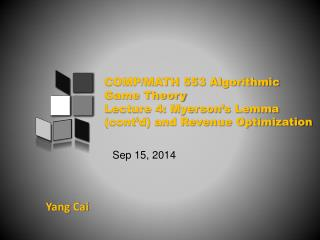DownloadDownload PresentationYang Cai

# Yang Cai

Télécharger la présentation## Yang Cai

- - - - - - - - - - - - - - - - - - - - - - - - - - - E N D - - - - - - - - - - - - - - - - - - - - - - - - - - -
##### Presentation Transcript

1. COMP/MATH 553 Algorithmic Game TheoryLecture 4: Myerson’s Lemma (cont’d) and Revenue Optimization Sep 15,2014 YangCai

2. An overview of today’s class Myerson’s Lemma (cont’d) Application of Myerson’s Lemma Revelation Principle Intro to Revenue Maximization

3. Myerson’s Lemma • [Myerson ’81 ] Fix a single-dimensional environment. • An allocation rule x is implementable if and only if it is monotone. • (b) If x is monotone, then there is a unique payment rule such that the sealed-bid mechanism (x, p) is DSIC [assuming the normalization that bi = 0 implies pi(b) = 0]. • (c) The payment rule in (b) is given by an explicit formula.

4. Application of Myerson’s Lemma

5. Single-item Auctions: Set-up Bidders v1 Item 1 Auctioneer … vi i vn … n • Allocation Rule: give the item to the highest bidder. • ✔ • Payment Rule: ?

6. Sponsored Search Auctions: Set-up Slots Bidders (advertisers) Auctioneer/Google v1 α1 1 1 … … αj vi i j αk vn … … n • Allocation Rule: allocate the slots greedily based on the bidders’ bids. • ✔ • Payment Rule: ? k

7. Revelation Principle

8. ? Q: Why DSIC? • It’s easy for the bidders to play. • Designer can predict the outcome with weakassumption on bidders’ behavior. • But sometimes first price auctions can be useful in practice. • Can non-DSICmechanisms accomplish things that DSIC mechanisms can’t?

9. Two assumptions about DSIC • Assumption (1): Everyparticipant in the mechanism has a dominant strategy, no matter what its private valuation is. • Assumption (2): This dominant strategy is direct revelation, where the participant truthfully reports all of its private information to the mechanism. • There are mechanisms that satisfy (1) but not (2). • Run Vickrey on bids × 2...

10. DSIC? • Assumption (1): Everyparticipant in the mechanism has a dominant strategy, no matter what its private valuation is. • Can relax (1)? but need stronger assumptions on the bidders’ behavior, e.g. Nash eq. or Bayes-Nash eq. • Relaxing (1) can give stronger results in certain settings. • DSIC is enough for most of the simple settings in this class. • Incomparable: Performance or Robustness?

11. Revelation Principle • Assumption 2: This dominant strategy is direct revelation, where the participant truthfully reports all of its private information to the mechanism. • Comes for “free”. • Proof: Simulation.

12. Revelation Principle Theorem (Revelation Principle): For every mechanism M in which every participant has a dominant strategy (no matter what its private information), there is an equivalent direct-revelation DSIC mechanism M′.

13. Revelation Principle • Same principle can be extended to other solution concept, e.g. Bayes Nash Eq. • The requirement of truthfulness is not what makes mechanism design hard... • It’s hard to find a desired outcome in a certain type of Equilibrium. • Changing the type of equilibrium leads to different theory of mechanism design.

14. REVENUE-OPTIMAL AUCTION

15. Welfare Maximization, Revisited • Why did we start with Welfare? • Obviously a fundamental objective, and has broad real world applications. (government, highly competitive markets) • For welfare, you have DSIC achieving the optimal welfare as if you know the values (single item, sponsored search, and even arbitrary settings (will cover in the future)) • Not true for many other objectives.

16. One Bidder + One Item • The only DSIC auctions are the “posted prices”. • If the seller posts a price of r, then the revenue is either r (if v ≥ r), or 0 (if v < r). • If we know v, we will set r = v. But v is private... • Fundamental issue is that, for revenue, different auctions do better on different inputs. • Requires a model to reason about tradeoffs between different inputs.

17. Bayesian Analysis/Average Case • Classical Model: pose a distribution over the inputs, and compare the expected performance. • A single-dimensional environment. • The private valuation vi of participant i is assumed to be drawn from a distribution Fi with density function fi with support contained in [0,vmax]. • We assume that the distributions F1, . . . , Fnare independent (not necessarily identical). • In practice, these distributions are typically derived from data, such as bids in past auctions. • The distributions F1 , . . . , Fnare known in advance to the mechanism designer. The realizations v1, . . . , vnof bidders’ valuations are private, as usual.

18. Solution for One Bidder + One Item • Expected revenue of a posted price r is r (1−F(r)) • When F is the uniform dist. on [0,1], optimal choice of r is ½ achieving revenue ¼. • The optimal posted price is also called the monopoly price.

19. Two Bidders + One Item • Two bidders’ values are drawn i.i.d. from U[0,1]. • Revenue of Vickrey’s Auction is the expectation of the min of the two random variables = 1/3. • What else can you do? Can try reserve price. • Vickrey with reserve at ½ gives revenue 5/12 > 1/3. • Can we do better?

20. Revenue-Optimal Auctions • [Myerson ’81 ] • Single-dimensional settings • Simple Revenue-Optimal auction